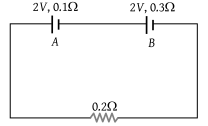The internal resistances of two cells shown are 0.1 Ω and 0.3 Ω. If R = 0.2 Ω, the potential difference across the cell(1) B will be zero

(2) A will be zero

(3) A and B will be 2V

(4) A will be > 2V and B will be < 2V

(1) Applying Kirchhoff law

$\left(2+2\right)=\left(0.1+0.3+0.2\right)i$$i=\frac{20}{3}A$

Hence potential difference across A

$=2-0.1×\frac{20}{3}=\frac{4}{3}V$ (less than 2V)

Potential difference across $B=2-0.3×\frac{20}{3}=0$

Difficulty Level:

• 47%
• 17%
• 15%
• 23%